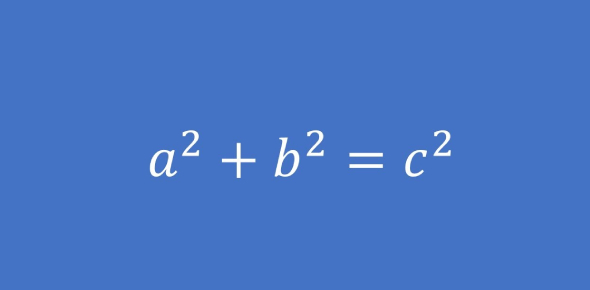# PRAXIS Core Math MCQ Test

10 Questions | Attempts: 13185SettingsMathematics is a wonderful subject, whether you feel it’s necessary for you or not. It’s a fundamental skill, and a good foundation of knowledge in it will make you look like a competent individual in any field of work that requires you to add, subtract, multiply or divide on the fly. In this Free Mini PRAXIS Core Math Test, we’ll be checking up on your basic maths knowledge to see how much you know!

• 1.
Of the five numbers listed below, which is the greatest?
• A.

0.00800

• B.

0.022

• C.

0.00004

• D.

0.0197

• E.

0.0202

• 2.
Natalie did 1/3 of her homework on Saturday and 1/5 more of her homework on Sunday. What is the ratio of the amount of homework she has completed to the amount of homework she has not completed?
• A.

3:5

• B.

7:8

• C.

8:7

• D.

7:15

• E.

8:15

• 3.
What digit is in the hundredths place of the number 978.0234?
• A.

2

• B.

3

• C.

4

• D.

7

• E.

8

• 4.
In a class of 25 students, 15 are boys. What percentage of the students in the class are girls?
• A.

10%

• B.

15%

• C.

25%

• D.

40%

• E.

60%

• 5.
A map is drawn with a scale of 4 inches representing 80 miles. How many inches on the map would represent 280 miles?
• A.

7

• B.

14

• C.

20

• D.

28

• E.

40

• 6.
Nicole spent \$60 on groceries the first week of July. The second week of July she spent \$75 on groceries. What was the percent increase in the amount spent by Nicole on groceries?
• A.

15%

• B.

20%

• C.

25%

• D.

40%

• E.

60%

• 7.
In which of the following are the two numbers equivalent?I.   1.2 and 1/2II.   0.8 and 0.800          III.  9 and 33
• A.

I

• B.

II

• C.

III

• D.

I and III

• E.

I, II, and III

• 8.
Which of the following is greater than 0.674?
• A.

2/3

• B.

0.0698

• C.

7/12

• D.

6.83%

• E.

11/16

• 9.
Which one of the following is NOT the same as a 3:7 ratio?
• A.

6:10

• B.

9:21

• C.

12:28

• D.

15:35

• E.

30:70

• 10.
Theodore bought a clock that is 18 centimeters tall. What is the height of the clock in millimeters?
• A.

0.18

• B.

1.8

• C.

180

• D.

1,800

• E.

18,000

## Related TopicsBack to top
×

Wait!
Here's an interesting quiz for you.